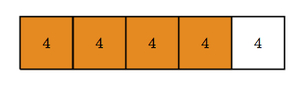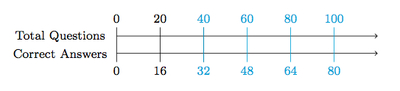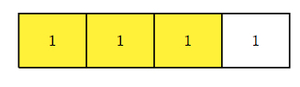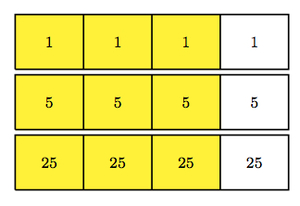# Exam scores

Alignments to Content Standards: 6.RP.A.3.c 6.RP.A.3

Alyssa took a math test with 20 questions and  she answered 16 questions correctly. In order get an A, she needs to answer at least 90 percent of the questions correctly.

1. Did Alyssa get an A on this math test? Explain.
2. How many questions would Alyssa need to answer correctly in order to get an A?
3. On the same exam, Alyssa's friend Jessie answered three times more questions correct than incorrect. Who got a higher percentage of the test questions correct, Alyssa or Jessie?

## IM Commentary

The goal of this task is to show how to apply ratio reasoning to calculate a percent. The numbers chosen make the arithmetic part of the task less demanding so that students can focus on the reasoning used to manipulate ratios. In order to do this task, students must know the meaning of percent, that is they need to know that a percent is a rate out of 100. In this case, it is the number of questions Alyssa would have answered correctly on a 100 question exam, assuming she maintains the same ratio of correct answers to questions.

Three different and important representations can be used to help calculate the percent of questions Alyssa answered correctly: tape diagrams, ratio tables, and double number lines. The teacher may wish to encourage students to work with these different representations. Students can practice the different representations in parts (b) and (c) of the task also. In the solution, direct reasoning with ratios is used in part (b) (and then translated into a very short ratio table) while a tape diagram is used in part (c) but all three representations are appropriate for these parts of the question as well.

## Solution

1. Alyssa's ratio of correct questions to total questions is 16 : 20. We can represent this with a tape diagram:The orange boxes show the 16 questions Alyssa answered correctly and the white box shows the 4 questions that she answered incorrectly. To see what percent of the questions Alyssa answered correctly, we can make a second tape diagram so that the 5 boxes add up to 100:Note that the ratio of correct to incorrect questions is the same in the two tape diagrams, as we can see since the parts line up with one another in the picture. The top tape diagram with 20's in the boxes shows that Alyssa got 80 percent of the questions correct (and 20 percent incorrect).

Another way to see the percent of questions Alyssa got correct is using a ratio table:

Questions Correct Questions on Exam
16 20
32 40
64 80
80 100

The ratios of questions answered correctly to questions on the exam are equivalent in each row of the table because we doubled the entries from the first row to get the second row. The entries are then doubled again for the third row and finally the first and third rows are added to get the fourth row. A similar technique can be used with a double number line:The given information is shown in black and the equivalent ratios derived from this are shown in blue.

2. In order to get an A on the exam, Alyssa needs a ratio of 90 : 100. Looking at the solutions in part (a), 100 is 5 times 20 so to see how many questions Alyssa needs to answer correctly out of 20 we can divide 90 by 5. Since 90 $\div$ 5 = 18, Alyssa needs to answer 18 questions correctly. The ratios 18 : 20 and and 90 : 100 are equivalent since both numbers are scaled by the same factor, 5, to get from the first pair of numbers to the second.

Using a ratio table, this information is shown below:

Questions Correct Questions on Exam
90 100
18 20

To obtain the second row from the first we multiply both entries by $\frac{1}{5}$.

3. Jessie answered 3 times as many questions correctly than she did incorrectly. This means that for every 1 question Jessie answered incorrectly, she answered 3 questions correctly. In other words, Jessie's ratio of correct answers to incorrect answers is 3 : 1 and this is pictured below:The exam had 20 questions so if we scale the numbers in the tape diagram by 5 we can see how many questions Jessie answered correctly and incorrectly on the exam. Then to see what percentage of the questions Jessie got right on the exam, we need to scale the numbers in the tape diagram a second time so that they add up to 100. In part (a) we saw that this also requires a scale factor of 5. Putting all of this together we have:Here we see that Jessie answered 75 percent of the questions correctly and 25 percent incorrectly. Alyssa did better on this exam than Jessie, getting 80 percent of the questions correct versus 75 percent.

Alternatively, we can present all of this information in a ratio table:

Questions Correct Questions Incorrect Total Questions
3 1 4
15 5 20
75 25 100

The first line of the table shows the given information, that Jessie answered three times as many questions correct than questions incorrect. The second row shows how many questions Jessie answered correctly and incorrectly on the exam. And the third line shows the percent of questions answered corretly and incorrectly.